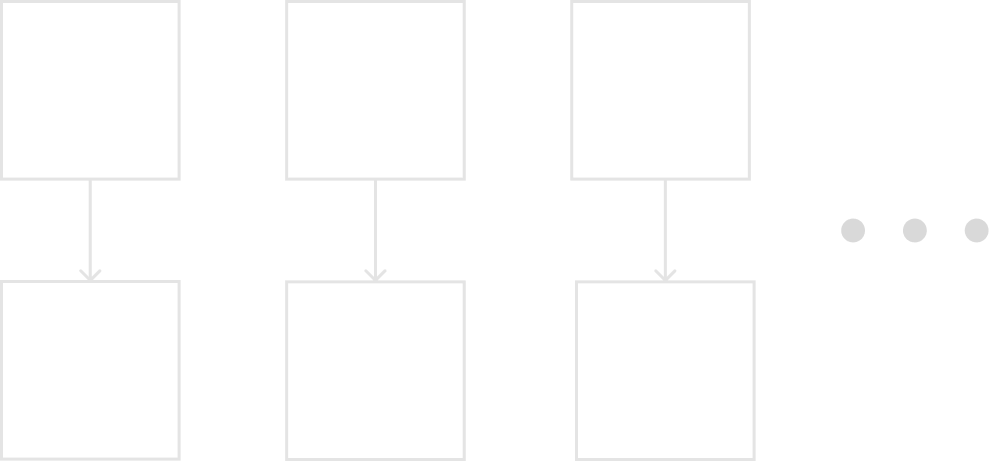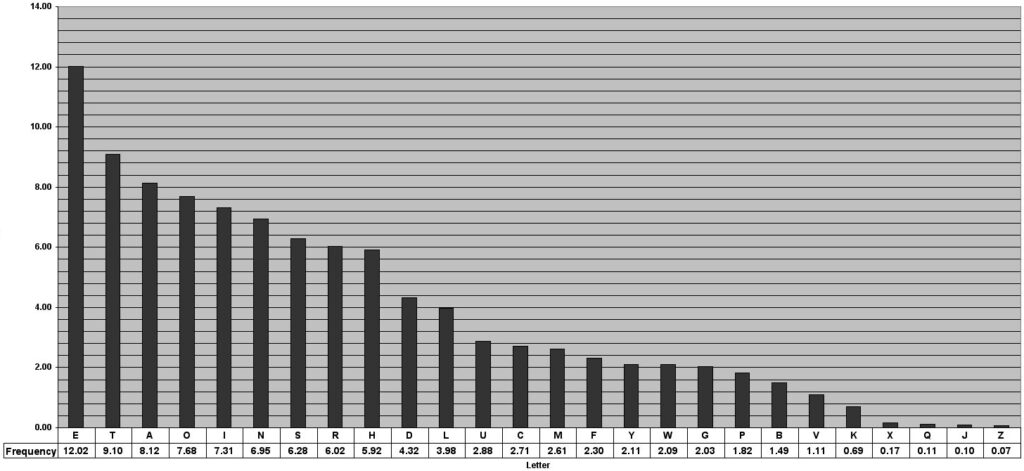Back To All

# The Substitution Cipher## The Substitution Cipher

We will discuss one of the simplest ciphers, the substitution cipher. This cipher has a lot of historical relevance being used many times, and is also a good starting point for cryptography and cryptanalysis. It is important to have read the What is Cryptology post because I will use concepts and notation discussed in the post.
The substitution cipher operates on letters. The idea of the substiution cipher is to replace every plaintext letter with a ciphertext letter. This is usually represented with a mapping shown in the diagram below:Thus in this example the plaintext ABBA would be encrypted as KDDK.

### Example

An important question to any cipher is the key to the cipher. As the key is the necessary component of any modern cryptosystem. So what is the key in a substitution cipher? For that, we will introduce two new notations for that, the plaintext alphabet and the ciphertext alphabet. The plaintext alphabet is what it sounds like, just the plain alphabet and the ciphertext alphabet is the key, ie what we are replacing each letter in the plaintext alphabet with. Here is an example:

 Plaintext Alphabet ABCDEFGHIJKLMNOPQRSTUVWXYZ Ciphertext Alphabet LIONABCDEFGHJKMPQRSTUVWXYZ

In creating this ciphertext I used the keyword “LION”, this is a common trick to use a word or phrase with no repeating letters as a keyword, and filling in the rest of the alphabet with the rest of the unused letters.
Here is an example of a message encrypted with this ciphertext alphabet:

thequickbrownfoxjumpsoverthelazydog

Encrypts to

tdaqueogirmwkbmxfujpsmvartdahlzynmc

## Code implementation

The implementation of cryptography is also a very important part, so I will always try to give you some kind of implementation. For this example it will be in C++:

```				```
#include <iostream>
#include <string>

using namespace std;

string encrypt(string ciphertextAlphabet, string plaintext, string ciphertext, int plaintextSize);

int main()
{
// initialize ciphertext alphabet
string ciphertextAlphabet = "lionabcdefghjkmpqrstuvwxyz";

// enter plaintext and initialize ciphertext
string plaintext = "thequickbrownfoxjumpsoverthelazydog";
string ciphertext = "";

// get length of message
int plaintextSize = plaintext.length();

ciphertext = encrypt(ciphertextAlphabet, plaintext, ciphertext, plaintextSize);

cout << "Encrypted Ciphertext:  " << ciphertext << endl;
}

string encrypt(string ciphertextAlphabet, string plaintext, string ciphertext, int plaintextSize)
{
int position = 0;

// loop until we reach end of the message
for (int i = 0; i < plaintextSize; i++)
{
// use the ASCII value of the letter, and subtract 97
position = plaintext[i] - 97;
ciphertext += ciphertextAlphabet[position];
}

return ciphertext;
}
```
```

This is a very simple implementation of the substitution cipher. If you want more advanced implementations feel free to check out my GitHub page for more.

## Attacks

Being a historical cipher, it has plenty of faults. Some of them may not be obvious at first though. So it’s important to ask “How can we attack the substitution cipher?”.

### Brute-force attack

The Brute-force attack or also called the exhaustive key attack is a common attack and is the baseline for all other attacks. How the brute-force attack works by trying every single possible key the cipher can have. In the substitution cipher case that means we need to try every possible ciphertext alphabet.
Thus it is important to step back and think about how the ciphertext alphabet is made. As we know, we need to make a mapping from the plaintext alphabet to the ciphertext alphabet so that every letter in the plaintext alphabet has another letter in the ciphertext alphabet. Then notice that to choose the first mapping, we have 26 choices of letters, then for the second mapping we have 25 letters to choose from, and so on.
Then to get a count for how many keys we need aka the keyspace, this is:

|k| = 26⋅25⋅24⋅23⋅22⋅…⋅2⋅1 = 26! (the ! is the notation for factorial)

Therefore, the keyspace is |k| = 26! ≈ 2^88 that is 309 septillion. To put in some kind of perspective, my computer can try ~1 Billion keys per second. Still, it would still take years for my computer to find the key for just this one example. But if you are really interested I have my own project in C++ on my GitHub that breaks the example above that uses LION as a keyword.
So the keyspace is too large for a brute-force attack to work, then you may be wondering “Why is this cipher considered very weak then?” Well, that is because as cryptanalysts we have a lot more tools than just brute-force.

### Letter frequency analysis

The letter frequency analysis attack will be very common for the historical ciphers we will cover in the future like substitution, Caesar, vigenere, etc. The letter frequency analysis attack uses the fact that languages, and in this case the english language does not equally use each of the letters in its alphabet. So some letters are used more than others, here is a chart to show this:We can notice that is the most common letter in the English alphabet, occurring ~12% of the time. Therefore, if we have an encrypted message and count how many times each letter occurs we should get something similar to this chart. If we notice the is showing up 12% of the time, we can assume that E in the plaintext alphabet maps to P in the ciphertext alphabet.
This would let us then make guesses of which mapping is what, and from there we can use other observations like double letters, and common letter combinations to further narrow down what the ciphertext alphabet could be. If you want to try this yourself, I found this tool online so I highly encourage you to try it out on your own.